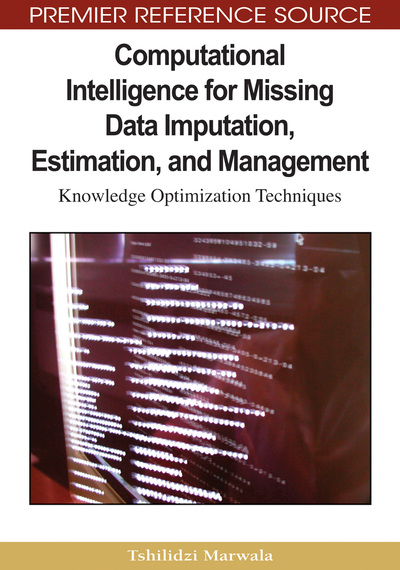# Estimation of Missing Data Using Neural Networks and Decision Trees

Tshilidzi Marwala
DOI: 10.4018/978-1-60566-336-4.ch011
OnDemand:
(Individual Chapters)
Available
\$37.50
No Current Special Offers

## Abstract

This chapter introduces a novel paradigm to impute missing data that combines a decision tree, autoassociative neural network (AANN) model and a principal component analysis-neural network (PCA-NN) based model. These models are designed to answer the crucial question of whether the optimization bounds actually matter. For each model, the decision tree is used to predict search bounds for a hybrid simulated annealing and genetic algorithm method that minimizes an error function derived from the respective model. The models’ ability to impute missing data is tested and then compared using HIV sero-prevalance data. Results indicate an average increase in accuracy of 13% with the AANN based model’s average accuracy increasing from 75.8% to 86.3% while that of the PCA-NN based model increasing from 66.1% to 81.6%.
Chapter Preview
Top

## Introduction

Missing data is a widely recognized problem affecting large databases that creates problems in many applications that depend on access to complete data records such as data visualization and reporting tools. This problem also limits data analysts interested in making policy decisions based on statistical inference from the data and thus estimating missing data is often invaluable as it preserves information and produces better, less biased estimates than simple techniques (Fogarty, 2006; Abdella, 2005; Nelwamondo, 2006) such as listwise deletion and mean-value substitution (Yansaneh, Wallace, & Marker, 1998; Allison, 2000).

Inferences made from available data for a certain applications depend on the completeness and quality of the data being used in the analysis. Thus, inferences made from a complete data are most likely to be more accurate than those made from incomplete data. Moreover, there are time critical applications, which require us to estimate or approximate the values of some missing variables that have to be supplied in relation to the values of other corresponding variables. Such situations may arise in system that uses a number of instruments, and in some cases one or more of the sensors used in the system fail. In such a situation, the value of the missing sensor has to be estimated within a short time and with great precision, and by taking into account of the values of the other sensors in the system. Approximation of the missing values in such situations requires the estimation of the missing values taking into account of the inter-relationships that exists amongst the values of other corresponding variables.

The neural network approach (Freeman and Skapura, 1991; Haykin, 1999), such as the one adopted by Abdella and Marwala (2005), involves an optimization process for the estimation of missing data. In earlier chapters, methods such as genetic algorithms, simulated annealing and particle swarm optimization are used without much regard to the optimization bounds (Michalewicz, 1996; Forrest, 1996; Banzhaf et al., 1998). Therefore, what is missing in this book is how to identify and incorporate optimization bounds in the estimation of missing data problem. This question necessarily requires an answer to the questions: What is the best method for identifying optimization bounds in the missing data problem? Is the incorporation of optimization bounds in the missing data problem significant? This chapter seeks to answer these questions.

Yu (2007) introduced a new non-monotone line search technique and combined it with the spectral projected gradient method for solving the bound constrained optimization problems. The results obtained showed that the identified global convergence and numerical tests are efficient. Yamada, Tanino, and Inuiguchi (2001) studied an optimization problem for minimizing a convex function over the weakly efficient set of a multi-objective programming problem.

Sun, Chen, and Li (2007) used C4.5 decision tree which was trained to diagnose faults in rotating machinery. The method was validated using six kinds of running states (normal or without any defect, unbalance, rotor radial rub, oil whirl, shaft crack and a simultaneous state of unbalance and radial rub), as simulated on a Bently Rotor Kit RK4. The results obtained showed that C4.5 has higher accuracy and needs less training time than back-propagation network. Kirchner, Tölle, and Krieter (2006) optimized a decision tree technique and successfully applied this to simulated sow herd datasets. Based on these successes, this chapter estimates optimization bounds using decision trees.

## Complete Chapter List

Search this Book:
Reset